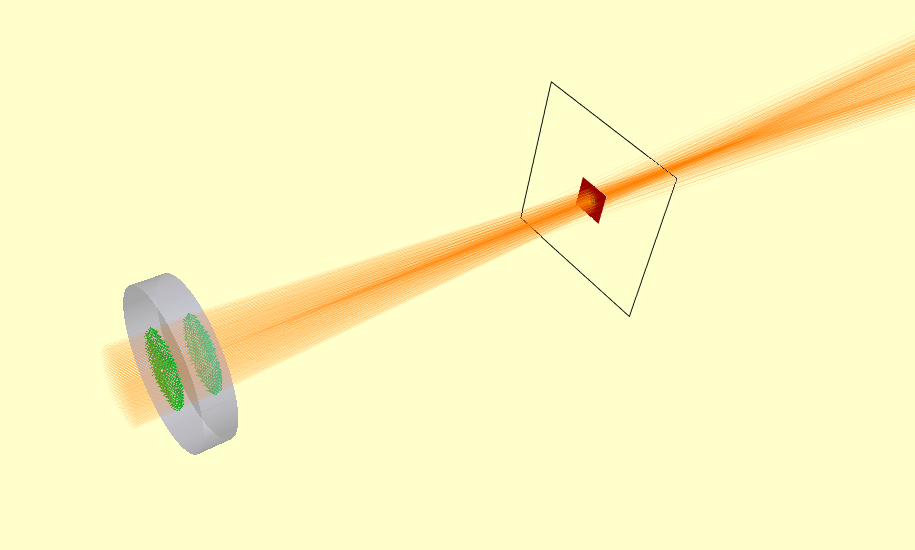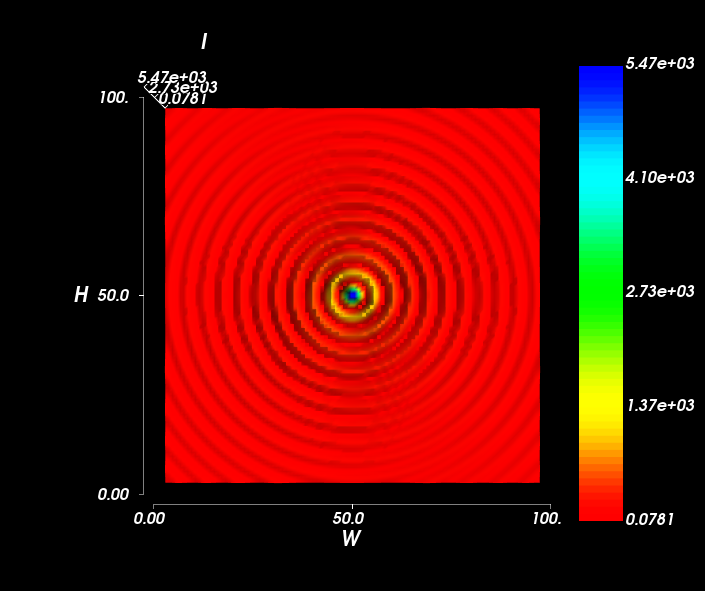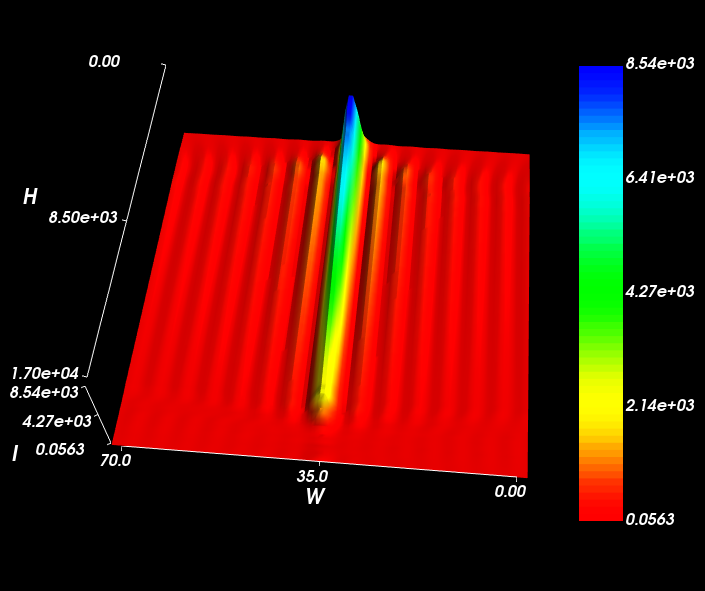Simulating a Bessel Beam¶

A Pseudo-bessel beam can be created using an Axicon. Where the rays from opposing sides of the axicon interact, they create a region of high intensity along the axis of the axicon. The interested properties of this beam is that it does not diffract. Modelling this with Raypier is straightforward. We use a CollimatedGaussianBeamSource to create the input wavefront.

from raypier.api import GeneralLens, AxiconFace, PlanarFace, OpticalMaterial, CircleShape,\
RayTraceModel, CollimatedGaussletSource, EFieldPlane, GaussletCapturePlane, IntensitySurface

from raypier.intensity_image import IntensityImageView

face1 = PlanarFace(z_height=0.0)

mat = OpticalMaterial(glass_name="N-BK7")

axicon = GeneralLens(name = "My Axicon",
centre = (0,0,0),
direction=(0,0,1),
shape=shape,
surfaces=[face1,
face2],
materials=[mat])

src = CollimatedGaussletSource(origin=(0.001,0,-5.0),
direction=(0,0,1),
wavelength=0.5,
beam_waist=10.0,
resolution=10,
max_ray_len=50.0,
display='wires',
opacity=0.2
)

capture = GaussletCapturePlane(centre=(0,0,13),
direction=(0,0,1),
width=5.0,
height=5.0)

field = EFieldPlane(centre=(0,0,13),
direction=(0,0,1),
detector=capture,
align_detector=True,
size=100,
width=2,
height=2)

image = IntensityImageView(field_probe=field)
surf = IntensitySurface(field_probe=field)

model = RayTraceModel(optics=[axicon],
sources=[src],
probes=[capture,field],
results=[image,surf])
model.configure_traits()

Here’s the model view.To get accurate results, turn up the resolution of the source object to about 30-40. Reduce the width of the EFieldPanel to ~0.1 to see the centre of the beam more clearly.

In the XY-plane, the characteristic Bessel rings are clear.Looking along the Z-axis (the optical axis), the constant width of the central beam is observed.